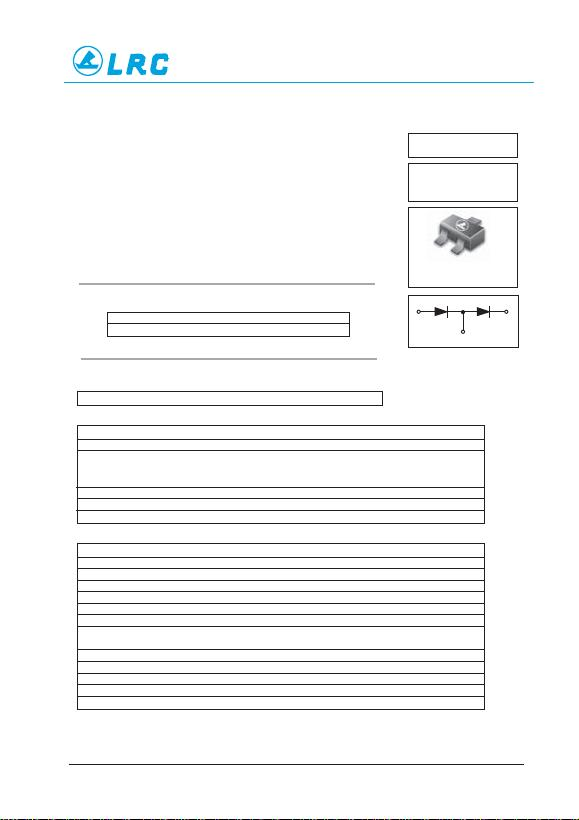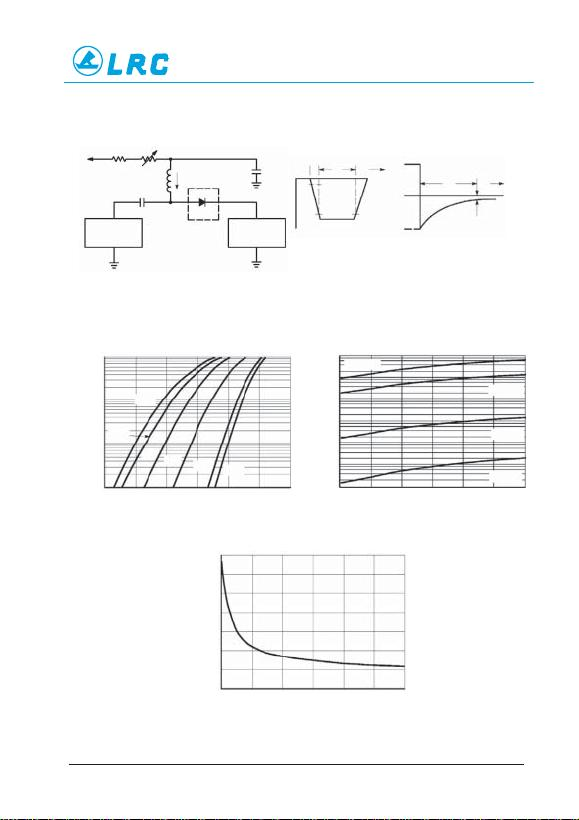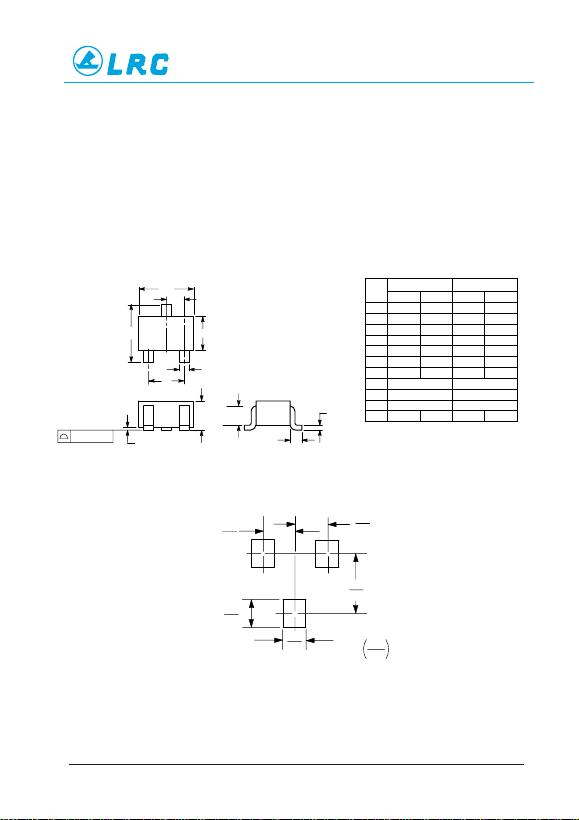LBAT54SWT1–1/3
1
3
2
DEVICE MARKING
LBAT54SWT1 = B8
MAXIMUM RATINGS (T J= 125°C unless otherwise noted)
Rating Symbol Value Unit
Reverse Voltage V R30 Volts
Forward Power Dissipation P F
@ T A= 25°C 200 mW
Derate above 25°C 1.6 mW/°C
Forward Current(DC) IF200Max mA
Junction Temperature T J125Max °C
Storage Temperature Range T stg –55 to +150 °C
ELECTRICAL CHARACTERISTICS (T A= 25°C unless otherwise noted)
Characteristic Symbol Min Typ Max Unit
Reverse Breakdown Voltage (I R= 10 µA) V(BR)R 30 Volts
Total Capacitance (V R= 1.0 V, f = 1.0 MHz) CT 7.6 10 pF
Reverse Leakage (V R= 25 V) I R 0.5 2.0 µAdc
Forward Voltage (I F= 0.1 mAdc) VF 0.22 0.24 Vdc
Forward Voltage (I F= 30 mAdc) VF 0.41 0.5 Vdc
Forward Voltage (I F= 100 mAdc) VF 0.52 1.0 Vdc
Reverse Recovery T ime
(I F= I R= 10 mAdc, I R(REC) = 1.0 mAdc, Figure 1) trr 5.0 ns
Forward Voltage (I F= 1.0 mAdc) VF 0.29 0.32 Vdc
Forward Voltage (I F= 10 mAdc) VF 0.35 0.40 Vdc
Forward Current (DC) IF 200 mAdc
Repetitive Peak Forward Current IFRM 300 mAdc
Non–Repetitive Peak Forward Current (t < 1.0 s) IFSM 600 mAdc
LBAT54SWT1
SOT–323 (SC–70)
These Schottky barrier diodes are designed for high speed switching
applications, circuit protection, and voltage clamping. Extremely low forward
voltage reduces conduction loss. Miniature surface mount package is excel-
lent for hand held and portable applications where space is limited.
• Extremely Fast Switching Speed
• Low Forward Voltage — 0.35 Volts (Typ) @ I F= 10 mAdc
30 VOLTS DUAL SERIES
SCHOTTKYAND BARRIER
DIODES
ORDERING INFORMATION
Device Package Shipping
LBAT54SWT1 SOT–323 3000/Tape & Reel
Preferred: devices are recommended choices for future use and best overall value.
3
CATHODE/ANODE
ANODE
1CATHODE
2
Dual Series Schottky
Barrier DiodesLBAT54SWT1–2/3
LBAT54SWT1
Notes: 1. A 2.0 k variable resistor adjusted for a Forward Current (I F) of 10mA.
Notes: 2. Input pulse is adjusted so I R(peak) is equal to 10mA.
Notes: 3. t p» t rr
Figure 1. Recovery Time Equivalent Test Circuit
100
10
1.0
0.1 0 0.1 0.2 0.3 0.4 0.5 0.6
150°C
T
A
= 150°C
T
A
= 125°C
T
A
= 85°C
T
A
= 25°C
0 5 10 15 20 25 30
1000
100
10
1.0
0.1
0.01
0.001
0 5 10 15 20 25 30
14
12
10
8
6
4
2
0
IF, FORWARD CURRENT (mA)
VF, FORWARD VOLTAGE (VOLTS)
Figure 2. Forward Voltage VR, REVERSE VOLTAGE (VOLTS)
Figure 3. Leakage Current
VR, REVERSE VOLTAGE (VOLTS)
Figure 4. Total Capacitance
IR, REVERSE CURRENT (µA)
125°C
85°C 25°C – 40°C –55°C
CTTOTAL CAPACITANCE (pF)
+10 V 2.0 k
820
100 µH0.1µF
D.U.T.
0.1 µF
50 OUTPUT
PULSE
GENERATOR
tr
50 INPUT
SAMPLING
OSCILLOSCOPE
tpt
10%
90%
IF
IR
trr t
iR(REC) = 1.0 mA
OUTPUT PULSE
(I F= I R= 10 mA; MEASURED
at i R(REC) = 1.0 mA)
INPUT SIGNAL
IF
VRLBAT54SWT1–3/3
0.7
1.9
0.028
0.65
0.025
0.65
0.025
inches
mm
0.075
0.035
0.9
NOTES:
1.
DIMENSION
I
NG AND TOLERANCING PER ANSI
Y14.5M, 1982.
2. CONTROLLING DIMENSION: INCH.
DIM MIN MAX MIN MA X
MILLIMETERSINCHES
A 0.071 0.087 1.80 2.20
K 0.017 REF 0.425 REF
L 0.026 BSC 0.650 BSC
N 0.028 REF 0.700 REF
S 0.079 0.095 2.00 2.40
B 0.045 0.053 1.15 1.35
C 0.032 0.040 0.80 1.00
D 0.012 0.016 0.30 0.40
G 0.047 0.055 1.20 1.40
H 0.000 0.004 0.00 0.10
J 0.004 0.010 0.10 0.25
CN
AL
D
G
SB
H
J
K
3
12
0.05 (0.002)
SC
-
70 / SOT
-
323